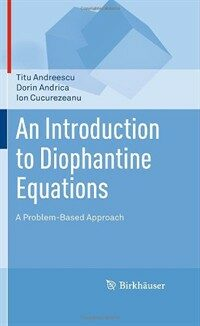> 상세정보

# 상세정보## An introduction to diophantine equations : a problem-based approach (2회 대출)

자료유형
단행본
개인저자
Andreescu, Titu, 1956-. Andrica, D. (Dorin). Cucurezeanu, Ion.
서명 / 저자사항
An introduction to diophantine equations : a problem-based approach / Titu Andreescu, Dorin Andrica, Ion Cucurezeanu.
발행사항
New York :   Birkhäuser,   c2010.
형태사항
xi, 345 p. ; 24 cm.
ISBN
9780817645489 (alk. paper) 0817645489 (alk. paper) 9780817645496 (e-ISBN) 0817645497 (e-ISBN)
요약
"This problem-solving book is an introduction to the study of Diophantine equations, a class of equations in which only integer solutions are allowed. The material is organized in two parts: Part I introduces the reader to elementary methods necessary in solving Diophantine equations, such as the decomposition method, inequalities, the parametric method, modular arithmetic, mathematical induction, Fermat's method of infinite descent, and the method of quadratic fields; Part II contains complete solutions to all exercises in Part I. The presentation features some classical Diophantine equations, including linear, Pythagorean, and some higher degree equations, as well as exponential Diophantine equations. Many of the selected exercises and problems are original or are presented with original solutions. [This book] is intended for undergraduates, advanced high school students and teachers, mathematical contest participants - including Olympiad and Putnam competitors - as well as readers interested in essential mathematics. The work uniquely presents unconventional and non-routine examples, ideas, and techniques."--From back cover.
내용주기
Diophantine equations -- Elementary methods for solving Diophantine equations -- Some classical Diophantine equations -- Pell-type equations -- Some advanced methods for solving Diophantine equations -- Solutions to exercises and problems -- Solutions to elementary methods for solving Diophantine equations -- Solutions to some classical Diophantine equations -- Solutions to Pell-type equations -- solutions to some advanced methods in solving Diophantine equations -- References -- Glossary -- Index.
서지주기
Includes bibliographical references (p. -330) and index.
일반주제명
Diophantine equations.
 000 00000cam u2200205 a 4500 001 000045926621 005 20180111144255 008 180111s2010 nyu b 001 0 eng d 010 ▼a 2010934856 020 ▼a 9780817645489 (alk. paper) 020 ▼a 0817645489 (alk. paper) 020 ▼a 9780817645496 (e-ISBN) 020 ▼a 0817645497 (e-ISBN) 035 ▼a (KERIS)REF000016839928 037 ▼a 978-0-8176-4548-9 ▼b Springer ▼n http://www.springerlink.com 040 ▼a NUI ▼c NUI ▼d RRR ▼d YDXCP ▼d BTCTA ▼d BAKER ▼d PBU ▼d TUU ▼d MRW ▼d DLC ▼d 211009 050 0 0 ▼a QA242 ▼b .A53 2010 082 0 4 ▼a 512.7/4 ▼2 23 084 ▼a 512.74 ▼2 DDCK 090 ▼a 512.74 ▼b A558i 100 1 ▼a Andreescu, Titu, ▼d 1956-. 245 1 3 ▼a An introduction to diophantine equations : ▼b a problem-based approach / ▼c Titu Andreescu, Dorin Andrica, Ion Cucurezeanu. 260 ▼a New York : ▼b Birkhäuser, ▼c c2010. 300 ▼a xi, 345 p. ; ▼c 24 cm. 504 ▼a Includes bibliographical references (p. -330) and index. 505 0 ▼a Diophantine equations -- Elementary methods for solving Diophantine equations -- Some classical Diophantine equations -- Pell-type equations -- Some advanced methods for solving Diophantine equations -- Solutions to exercises and problems -- Solutions to elementary methods for solving Diophantine equations -- Solutions to some classical Diophantine equations -- Solutions to Pell-type equations -- solutions to some advanced methods in solving Diophantine equations -- References -- Glossary -- Index. 520 ▼a "This problem-solving book is an introduction to the study of Diophantine equations, a class of equations in which only integer solutions are allowed. The material is organized in two parts: Part I introduces the reader to elementary methods necessary in solving Diophantine equations, such as the decomposition method, inequalities, the parametric method, modular arithmetic, mathematical induction, Fermat's method of infinite descent, and the method of quadratic fields; Part II contains complete solutions to all exercises in Part I. The presentation features some classical Diophantine equations, including linear, Pythagorean, and some higher degree equations, as well as exponential Diophantine equations. Many of the selected exercises and problems are original or are presented with original solutions. [This book] is intended for undergraduates, advanced high school students and teachers, mathematical contest participants - including Olympiad and Putnam competitors - as well as readers interested in essential mathematics. The work uniquely presents unconventional and non-routine examples, ideas, and techniques."--From back cover. 650 0 ▼a Diophantine equations. 700 1 ▼a Andrica, D. ▼q (Dorin). 700 1 ▼a Cucurezeanu, Ion. 945 ▼a KLPA

### 소장정보

No. 소장처 청구기호 등록번호 도서상태 반납예정일 예약 서비스
No. 1 소장처 청구기호 512.74 A558i 등록번호 121242997 도서상태 대출가능 반납예정일 예약 서비스

### 컨텐츠정보

#### 티투 앤드레스쿠(지은이)

루마니아의 티미소아라 웨스트 대학교에서 ‘디오판틴 해석과 응용에 관한 연구’로 박사학위를 받았다. 앤드레스쿠 교수는 현재 댈러스의 텍사스 대학교에서 강의하고 있으며, 1998년부터 2003년까지 미국 수학회의 AMC 디렉터이자 미국 수학올림피아드 위원회의 위원장이었고, 1993년부터 2002년까지 10년간 국제 수학 올림피아드 미국 대표팀의 코치, 1995년부터 2002년까지 미국 수학 올림피아드 여름학교 디렉터, 1995년부터 2002년까지 국제 수학 올림피아드 미국 대표팀의 수장이었다. 2002년에는 국제 수학 올림피아드 위원회 고문으로 선출되어 세계에서 가장 권위 있는 수학 시험을 주관했다. 2006년부터 ‘경외로운 수학’ 여름 학교 프로그램(AMSP: AwesomeMath Summer Program)을 공동 설립하고 현재까지 운영해오고 있다. 1994년에는 미국 수학회로부터 최고의 고교수학 교사에게 주어지는 Edyth May Sliffe 상을 받았고, 1994년 홍콩에서 열린 국제 수학 올림피아드에서 우수한 성적을 낸 미국 대표팀을 훈련시킨 공로로 1995년 미국 수학회로부터 감사패를 받았다. 티투 교수의 수많은 책과 문제집들은 전 세계적으로 유명하다.

#### 목차

`Diophantine Equations -- Elementary Methods for Solving Diophantine Equations -- Some Classical Diophantine Equations -- Pell-Type Equations -- Some Advanced Methods for Solving Diophantine Equations -- Solutions to Exercises and Problems -- Solutions to Elementary Methods for Solving Diophantine Equations -- Solutions to Some Classical Diophantine Equations -- Solutions to Pell-Type Equations -- Solutions to Some Advanced Methods in Solving Diophantine Equations.`

김대수 (2022)

민만식 (2022)